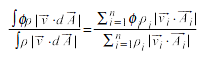# Calculating Mass-Weighted Average

#### How do I calculate the mass-weighted average of a scalar through a surface?

Mass weighted average can be obtained from the combined use of the function editor and two separate integrations. The mass weighted average is usually defined as:Thus, it can be computed by dividing the summation of the value of the selected variable multiplied by the absolute value of the dot product of the facet area and momentum vectors by the summation of the absolute value of the dot product of the facet area and momentum vectors. If the normal to your surface of interest in known by FieldView, you’ll be able to compute Int(V dot N) for the current vector. Thus, the mass weighted average will be obtained by:

• Creating a new vector, using the function editor, multiplying your scalar of interest by the momentum vector.
• Integrating this vector on your surface of interest. The result of that integration is given by FieldView under the “Int(V dot N)” field.
• Integrating the momentum vector in the same way.
• Dividing the 1st result by the second one.

NOTE: in the case of a boundary surface, the normal may not be known by FieldView, because the correct direction has not been provided by the solver. FieldView then reports the following to your terminal:

WARNING: boundary clockness flag was set, but boundary faces had inconsistent clockness. Therefore, the boundary face normals will not be used. Boundary surface integrals with normals will not be available.

In such a case, you can still calculate mass weighted average in the same way for planar surfaces such as Coordinate Surfaces or Cutting Planes.